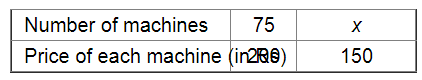# Raghu has enough money to buy 75 machines worth Rs 200 each.`
Question:

Raghu has enough money to buy 75 machines worth Rs 200 each. How many machines can he buy if he gets a discount of Rs 50 on each machine?

Solution:

Let x be the number of machines he can buy if a discount of Rs. 50 is offered on each machine.Since Raghu is getting a discount of Rs 50 on each machine, the cost of each machine will get decreased by Rs 50 .

If the price of a machine is less, he can buy more number of machines.

It is a case of inverse variation. Therefore, we have:

$75 \times 200=x \times 150$

$\Rightarrow x=\frac{75 \times 200}{150}$

$=\frac{15000}{150}$

$=100$

$\therefore$ The number of machines he can buy is 100 .